Tamil Nadu Board of Secondary EducationHSC Science Class 12

# Tamil Nadu Board Samacheer Kalvi solutions for Class 12th Physics Volume 1 and 2 Answers Guide chapter 4 - Electromagnetic Induction And Alternating Current [Latest edition]

#### Chapters## Solutions for Chapter 4: Electromagnetic Induction And Alternating Current

Below listed, you can find solutions for Chapter 4 of Tamil Nadu Board of Secondary Education Tamil Nadu Board Samacheer Kalvi for Class 12th Physics Volume 1 and 2 Answers Guide.

Evaluation
Evaluation [Pages 257 - 264]

### Tamil Nadu Board Samacheer Kalvi solutions for Class 12th Physics Volume 1 and 2 Answers Guide Chapter 4 Electromagnetic Induction And Alternating Current Evaluation [Pages 257 - 264]

#### Multiple Choice Questions

Evaluation | Q I. 1. | Page 257

An electron moves on a straight-line path XY as shown in the figure. The coil abcd is adjacent to the path of the electron. What will be the direction of the current, if any, induced in the coil?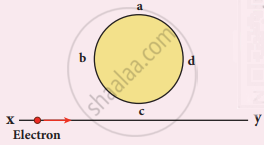• The current will reverse its direction as the electron goes past the coil

• No current will be induced

• abcd

Evaluation | Q I. 2. | Page 258

A thin semi-circular conducting ring (PQR) of radius r is falling with its plane vertical in a horizontal magnetic field B, as shown in the figure.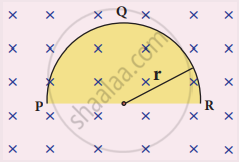The potential difference developed across the ring when its speed v , is

• Zero

• ("Bv"pi"r"^2)/2 and P is at higher potential

• πrBv and R is at higher potential

• 2rBv and R is at higher potential

Evaluation | Q I. 3. | Page 258

The flux linked with a coil at any instant t is given by ΦB = − 10t2 - 50t + 250. The induced emf at t = 3 s is

• - 190 V

• - 10 V

• 10 V

• 190 V

Evaluation | Q I. 4. | Page 258

When the current changes from +2A to −2A in 0.05 s, an emf of 8 V is induced in a coil. The co-efficient of self-induction of the coil is

• 0.2 H

• 0.4 H

• 0.8 H

• 0.1 H

Evaluation | Q I. 5. | Page 258

The current i flowing in a coil varies with time as shown in the figure. The variation of induced emf with time would be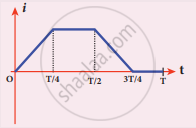•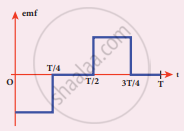•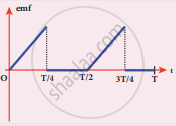•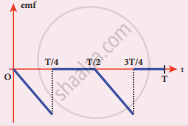•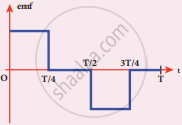Evaluation | Q I. 6. | Page 259

A circular coil with a cross-sectional area of 4 cm2 has 10 turns. It is placed at the centre of a long solenoid that has 15 turns/cm and a cross-sectional area of 10 cm2. The axis of the coil coincides with the axis of the solenoid. What is their mutual inductance?

• 7.54 µH

• 8.54 µH

• 9.54 µH

• 10.54 µH

Evaluation | Q I. 7. | Page 259

In a transformer, the number of turns in the primary and the secondary are 410 and 1230 respectively. If the current in primary is 6A, then that in the secondary coil is

• 2 A

• 18 A

• 12 A

• 1 A

Evaluation | Q I. 8. | Page 259

A step-down transformer reduces the supply voltage from 220 V to 11 V and increase the current from 6 A to 100 A. Then its efficiency is

• 1.2

• 0.83

• 0.12

• 0.9

Evaluation | Q I. 9. | Page 259

In an electrical circuit, R, L, C, and AC voltage sources are all connected in series. When L is removed from the circuit, the phase difference between the voltage and current in the circuit is π/3. Instead, if C is removed from the circuit, the phase difference is again π/3. The power factor of the circuit is

• 1/2

• 1/sqrt2

• 1

• sqrt3/2

Evaluation | Q I. 10. | Page 259

In a series RL circuit, the resistance and inductive reactance are the same. Then the phase difference between the voltage and current in the circuit is

• pi/4

• pi/2

• pi/6

• zero

Evaluation | Q I. 11. | Page 259

In a series resonant RLC circuit, the voltage across 100 Ω resistor is 40 V. The resonant frequency ω is 250 rad/s. If the value of C is 4 µF, then the voltage across L is

• 600 V

• 4000 V

• 400 V

• 1 V

Evaluation | Q I. 12. | Page 259

An inductor 20 mH, a capacitor 50 μF and a resistor 40Ω are connected in series across a source of emf V = 10 sin 340 t. The power loss in AC circuit is

• 0.76 W

• 0.89 W

• 0.46 W

• 0.67 W

Evaluation | Q I. 13. | Page 259

The instantaneous values of alternating current and voltage in a circuit are

i = 1/sqrt2 sin 100π A and

v = 1/sqrt2 sin (100 pi"t" + pi/3)"V".

The average power in watts consumed in the circuit is

• 1/4

• sqrt3/4

• 1/2

• 1/8

Evaluation | Q I. 14. | Page 259

In an oscillating LC circuit, the maximum charge on the capacitor is Q. The charge on the capacitor when the energy is stored equally between the electric and magnetic fields is

• "Q"/2

• "Q"/sqrt3

• "Q"/sqrt2

• Q

Evaluation | Q I. 15. | Page 260

20/pi^2H inductor is connected to a capacitor of capacitance C. The value of C in order to impart maximum power at 50 Hz is

• 50 μF

• 0.5 μF

• 500 μF

• 5 μF

Evaluation | Q II. 1. | Page 260

What is meant by electromagnetic induction?

Evaluation | Q II. 2. | Page 260

State Faraday’s laws of electromagnetic induction.

Evaluation | Q II. 3. | Page 260

State Lenz’s law.

Evaluation | Q II. 4. | Page 260

State Fleming’s right-hand rule.

Evaluation | Q II. 5 | Page 260

How is Eddy current produced? How do they flow in a conductor?

Evaluation | Q II. 6. | Page 260

Mention the ways of producing induced emf.

Evaluation | Q II. 7. | Page 260

What for an inductor is used? Give some examples.

Evaluation | Q II. 8. | Page 260

What do you mean by self-induction?

Evaluation | Q II. 9. | Page 260

What is meant by mutual induction?

Evaluation | Q II. 10. | Page 260

Give the principle of AC generator.

Evaluation | Q II. 11. | Page 260

List out the advantages of stationary armature-rotating field system of AC generator.

Evaluation | Q II. 12. | Page 260

What are step-up and step-down transformers?

Evaluation | Q II. 13. | Page 260

Define average value of an alternating current.

Evaluation | Q II. 14. | Page 260

How will you define RMS value of an alternating current?

Evaluation | Q II. 15. | Page 260

What are phasors?

Evaluation | Q II. 16. | Page 260

Define electric resonance.

Evaluation | Q II. 17. | Page 260

What do you mean by resonant frequency?

Evaluation | Q II. 18. | Page 260

How will you define Q-factor?

Evaluation | Q II. 19. | Page 260

What is meant by wattles current?

Evaluation | Q II. 20. | Page 260

Give any one definition of power factor.

Evaluation | Q II. 21. | Page 260

What are LC oscillations?

Evaluation | Q III. 1. | Page 260

Establish the fact that the relative motion between the coil and the magnet induces an emf in the coil of a closed circuit.

Evaluation | Q III. 2. | Page 260

Give an illustration of determining direction of induced current by using Lenz’s law.

Evaluation | Q III. 3. | Page 260

Show that Lenz’s law is in accordance with the law of conservation of energy.

Evaluation | Q III. 4. | Page 260

Obtain an expression for motional emf from Lorentz force.

Evaluation | Q III. 5. | Page 260

Give the uses of Foucault current.

Evaluation | Q III. 6. | Page 260

Define self-inductance of a coil interms of

1. magnetic flux and
2. induced emf
Evaluation | Q III. 7. | Page 260

How will you define the unit of inductance?

Evaluation | Q III. 8. | Page 260

What do you understand by self-inductance of a coil?

Evaluation | Q III. 8. | Page 260

Give the physical significance of self-inductance of a coil.

Evaluation | Q III. 9. | Page 260

Assuming that the length of the solenoid is large when compared to its diameter, find the equation for its inductance.

Evaluation | Q III. 10. | Page 261

An inductor of inductance L carries an electric current i. How much energy is stored while establishing the current in it?

Evaluation | Q III. 11. | Page 261

Show that the mutual inductance between a pair of coils is same (M12 = M21).

Evaluation | Q III. 12. | Page 261

How will you induce an emf by changing the area enclosed by the coil?

Evaluation | Q III. 13. | Page 261

Show mathematically that the rotation of a coil in a magnetic field over one rotation induces an alternating emf of one cycle.

Evaluation | Q III. 14. | Page 261

Elaborate the standard construction details of AC generator.

Evaluation | Q III. 15. | Page 261

Explain the working of a single-phase AC generator with necessary diagram.

Evaluation | Q III. 16. | Page 261

How are the three different emfs generated in a three-phase AC generator? Show the graphical representation of these three emfs.

Evaluation | Q III. 17. | Page 261

Explain the construction of transformer.

Evaluation | Q III. 17. | Page 261

Explain the working of the transformer.

Evaluation | Q III. 18. | Page 261

Mention the various energy losses in a transformer.

Evaluation | Q III. 19. | Page 261

Give the advantage of AC in long distance power transmission with an illustration.

Evaluation | Q III. 20. | Page 261

Find out the phase relationship between voltage and current in a pure inductive circuit.

Evaluation | Q III. 21. | Page 261

Derive an expression for phase angle between the applied voltage and current in a series RLC circuit.

Evaluation | Q III. 22. | Page 261

Define inductive reactance and give its units.

Evaluation | Q III. 22. | Page 261

Define capacitive reactance. Give its units.

Evaluation | Q III. 23. | Page 261

Obtain an expression for average power of AC over a cycle. Discuss its special cases.

Evaluation | Q III. 24. | Page 261

Explain the generation of LC oscillations in a circuit containing an inductor of inductance L and a capacitor of capacitance C.

Evaluation | Q III. 25. | Page 261

Show that the total energy is conserved during LC oscillations.

Evaluation | Q III. 26. | Page 261

Prove that energy is conserved during electromagnetic induction.

#### Numerical problems

Evaluation | Q IV. 1. | Page 261

A square coil of side 30 cm with 500 turns is kept in a uniform magnetic field of 0.4 T. The plane of the coil is inclined at an angle of 30° to the field. Calculate the magnetic flux through the coil.

Evaluation | Q IV. 2. | Page 261

A straight metal wire crosses a magnetic field of flux 4 mWb in a time 0.4 s. Find the magnitude of the emf induced in the wire.

Evaluation | Q IV. 3. | Page 261

The magnetic flux passing through a coil perpendicular to its plane is a function of time and is given by OB = (2t3 + 4t2 + 8t + 8) Wb. If the resistance of the coil is 5 Ω, determine the induced current through the coil at a time t = 3 second.

Evaluation | Q IV. 4. | Page 261

A closely wound circular coil of radius 0.02 m is placed perpendicular to the magnetic field. When the magnetic field is changed from 8000 T to 2000 T in 6 s, an emf of 44 V is induced in it. Calculate the number of turns in the coil.

Evaluation | Q IV. 5. | Page 262

A rectangular coil of area 6 cm2 having 3500 turns is kept in a uniform magnetic field of 0.4 T. Initially, the plane of the coil is perpendicular to the field and is then rotated through an angle of 180°. If the resistance of the coil is 35 Ω, find the amount of charge flowing through the coil.

Evaluation | Q IV. 6. | Page 262

An induced current of 2.5 mA flows through a single conductor of resistance 100 Ω. Find out the rate at which the magnetic flux is cut by the conductor.

Evaluation | Q IV. 7. | Page 262

A fan of metal blades of length 0.4 m rotates normal to a magnetic field of 4 x 10-3 T. If the induced emf between the centre and edge of the blade is 0.02 V, determine the rate of rotation of the blade.

Evaluation | Q IV. 8. | Page 262

A bicycle wheel with metal spokes of 1 m long rotates in Earth’s magnetic field. The plane of the wheel is perpendicular to the horizontal component of Earth’s field of 4 x 10-5 T. If the emf induced across the spokes is 31.4 mV, calculate the rate of revolution of the wheel.

Evaluation | Q IV. 9. | Page 262

Determine the self-inductance of 4000 turn air-core solenoid of length 2m and diameter 0.04 m.

Evaluation | Q IV. 10. | Page 262

A coil of 200 turns carries a current of 4 A. If the magnetic flux through the coil is 6 x 10-5 Wb, find the magnetic energy stored in the medium surrounding the coil.

Evaluation | Q IV. 11. | Page 262

A 50 cm long solenoid has 400 turns per cm. The diameter of the solenoid is 0.04 m. Find the magnetic flux linked with each turn when it carries a current of 1 A.

Evaluation | Q IV. 12. | Page 262

A coil of 200 turns carries a current of 0.4 A. If the magnetic flux of 4 mWb is linked with each turn of the coil, find the inductance of the coil.

Evaluation | Q IV. 13. | Page 262

Two air core solenoids have the same length of 80 cm and same cross–sectional area 5 cm2. Find the mutual inductance between them if the number of turns in the first coil is 1200 turns and that in the second coil is 400 turns.

Evaluation | Q IV. 14. | Page 262

A long solenoid having 400 turns per cm carries a current 2A. A 100 turn coil of cross-sectional area 4 cm2 is placed co-axially inside the solenoid so that the coil is in the field produced by the solenoid. Find the emf induced in the coil if the current through the solenoid reverses its direction in 0.04 sec.

Evaluation | Q IV. 15. | Page 262

A 200 turn circular coil of radius 2 cm is placed co-axially within a long solenoid of 3 cm radius. If the turn density of the solenoid is 90 turns per cm, then calculate mutual inductance of the coil and the solenoid.

Evaluation | Q IV. 16. | Page 262

The solenoids S1 and S2 are wound on an iron-core of relative permeability 900. The area of their cross-section and their length are the same and are 4 cm2 and 0.04 m, respectively. If the number of turns in S1 is 200 and that in S2 is 800, calculate the mutual inductance between the coils. The current in solenoid 1 is increased from 2A to 8A in 0.04 second. Calculate the induced emf in solenoid 2.

Evaluation | Q IV. 17. | Page 263

A step-down transformer connected to the main supply of 220 V is used to operate 11V,88W lamp. Calculate

1. Voltage transformation ratio and
2. Current in the primary
Evaluation | Q IV. 18. | Page 263

A 200V/120V step-down transformer of 90% efficiency is connected to an induction stove of resistance 40 Ω. Find the current drawn by the primary of the transformer.

Evaluation | Q IV. 19. | Page 263

The 300 turn primary of a transformer has resistance 0.82 Ω and the resistance of its secondary of 1200 turns is 6.2 Ω. Find the voltage across the primary if the power output from the secondary at 1600V is 32 kW. Calculate the power losses in both coils when the transformer efficiency is 80%

Evaluation | Q IV. 20. | Page 263

Calculate the instantaneous value at 60°, average value and RMS value of an alternating current whose peak value is 20 A.

#### Conceptual Questions

Evaluation | Q V. 1. | Page 263

A graph between the magnitude of the magnetic flux linked with a closed-loop and time is given in the figure. Arrange the regions of the graph in ascending order of the magnitude of induced emf in the loop.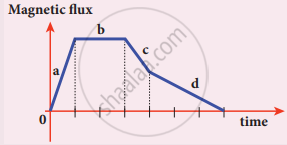Evaluation | Q V. 2. | Page 263

Using Lenz’s law, predict the direction of induced current in conducting rings 1 and 2 when the current in the wire is steadily decreasing.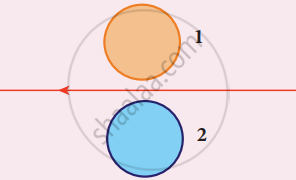Evaluation | Q V. 3. | Page 263

A flexible metallic loop abcd in the shape of a square is kept in a magnetic field with its plane perpendicular to the field. The magnetic field is directed into the paper normally. Find the direction of the induced current when the square loop is crushed into an irregular shape as shown in the figure.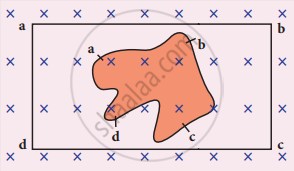Evaluation | Q V. 4. | Page 264

Predict the polarity of the capacitor in a closed circular loop when two bar magnets are moved as shown in the figure.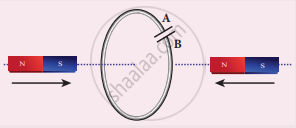Evaluation | Q V. 5. | Page 264

In a series LC circuit, the voltages across L and C are 180° out of phase. Is it correct? Explain.

Evaluation | Q V. 6. | Page 264

When does power factor of a series RLC circuit become maximum?

## Solutions for Chapter 4: Electromagnetic Induction And Alternating Current

Evaluation## Tamil Nadu Board Samacheer Kalvi solutions for Class 12th Physics Volume 1 and 2 Answers Guide chapter 4 - Electromagnetic Induction And Alternating Current

Shaalaa.com has the Tamil Nadu Board of Secondary Education Mathematics Class 12th Physics Volume 1 and 2 Answers Guide Tamil Nadu Board of Secondary Education solutions in a manner that help students grasp basic concepts better and faster. The detailed, step-by-step solutions will help you understand the concepts better and clarify any confusion. Tamil Nadu Board Samacheer Kalvi solutions for Mathematics Class 12th Physics Volume 1 and 2 Answers Guide Tamil Nadu Board of Secondary Education 4 (Electromagnetic Induction And Alternating Current) include all questions with answers and detailed explanations. This will clear students' doubts about questions and improve their application skills while preparing for board exams.

Further, we at Shaalaa.com provide such solutions so students can prepare for written exams. Tamil Nadu Board Samacheer Kalvi textbook solutions can be a core help for self-study and provide excellent self-help guidance for students.

Concepts covered in Class 12th Physics Volume 1 and 2 Answers Guide chapter 4 Electromagnetic Induction And Alternating Current are Electromagnetic Induction, Self–Induction, Transformers, Alternating Currents, Eddy Currents, Oscillation in LC Circuits, Methods of Producing Induced Emf, A.C. Generator, Power in AC Circuit.

Using Tamil Nadu Board Samacheer Kalvi Class 12th Physics Volume 1 and 2 Answers Guide solutions Electromagnetic Induction And Alternating Current exercise by students is an easy way to prepare for the exams, as they involve solutions arranged chapter-wise and also page-wise. The questions involved in Tamil Nadu Board Samacheer Kalvi Solutions are essential questions that can be asked in the final exam. Maximum Tamil Nadu Board of Secondary Education Class 12th Physics Volume 1 and 2 Answers Guide students prefer Tamil Nadu Board Samacheer Kalvi Textbook Solutions to score more in exams.

Get the free view of Chapter 4, Electromagnetic Induction And Alternating Current Class 12th Physics Volume 1 and 2 Answers Guide additional questions for Mathematics Class 12th Physics Volume 1 and 2 Answers Guide Tamil Nadu Board of Secondary Education, and you can use Shaalaa.com to keep it handy for your exam preparation.